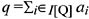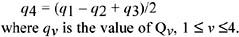Consider a population I of individuals and a database {ri: i I} of individual records which contain identifying (or key) attributes (e.g., name, SSN, tax-code), and descriptive attributes, which may be both qualitative (e.g., sex) and quantitative (e.g., salary). A category predicate is an arbitrary condition on descriptive attributes built up with the logical connectives (conjunction, disjunction and negation).

A count query Q is a question such as:

What is the number of individuals whose descriptive attributes satisfy condition P?

where P is a category predicate. If by I[Q] we denote the subset of I selected by P, the value of Q is the number of individuals in I[Q]; that is, |I[Q]|.

A sum query Q on a quantitative descriptive attribute a is a question such as:

What is the sum of a over the individuals whose descriptive attributes satisfy condition P?

where P is a category predicate involving descriptive attributes other than a. The attribute a is called the summary attribute of the query Q. If by I[Q] we denote the subset of I selected by P, the value of Q, denoted by q, is the sum of the values ai of a for all individuals i in I[Q]; that is,Note that, if the category predicate of an additive query Q is logically inconsistent, then I[Q] = Ø and the value of Q is taken to be zero. Such as query will be referred to as a null query.

Example 1: Consider the following database

name

sex

age

salary

John

M

25

2.0

Andrea

M

30

3.0

Mike

M

30

2.5

Mary

F

35

3.7

Helen

F

40

3.0

Anna

F

55

3.8

The following are four examples of sum queries on salary:

• Q1: What is the sum of the salaries of individuals with (sex = M)?

• Q2: What is the sum of the salaries of individuals with (sex = F)?

• Q3: What is the sum of the salaries of individuals with (age < 50)?

• Q4: What is the sum of the salaries of individuals with (age > 50)?

Suppose that our database contains a descriptive attribute a which is confidential. We saw in the previous section some sensitivity criteria; we now apply some of them to state precisely what queries are considered sensitive. A count query Q whose category predicate contains a is considered sensitive according to the n threshold rule if |I[Q]| n. Consider now a sum query Q with summary attribute a which is of numeric type. Let us distinguish two cases depending on whether the domain of a is the set of reals or integers, or is the set of nonnegative reals or integers. In the former case, we apply the n threshold criterion so that Q is sensitive if |I[Q]| n. In the latter case, we can apply either the n threshold criterion or the (n, k%) dominance rule. The security problem for additive queries can be stated as follows: Given a sensitivity criterion, what measures suffice to avoid disclosing values of sensitive queries? The following two examples on sum queries show that memory-less control methods (which only refuse to answer sensitive queries) are not secure.

Example 1 (continued): Assume that salary is a confidential attribute and that the sensitivity criterion in use is the n threshold criterion with n = 2. Thus, a sum query Q on salary is considered sensitive if |I[Q]| 2. Consider now the abovementioned queries Q1, Q2, Q3 and Q4; then Q1, Q2 and Q3 are not sensitive, and Q4 is sensitive. Suppose that Q1, Q2 and Q3 were answered and Q4 was left unanswered; nevertheless, the value of Q4 can be simply computed from the values of Q1, Q2 and Q3 by subtracting the answer (14.2) to Q3 from the sum (18.0) of the answers to Q1 and Q2.

Example 2: Consider the following database

name

department

salary

e1

Direction

10.05

e2

Direction

3.0

e3

Direction

1.95

e4

4.0

e5

2.55

e6

2.45

e7

Services

3.0

e8

Services

2.0

e9

Services

1.0

e10

Marketing

1.5

Again assume that salary is confidential and that the (2, 80%) dominance criterion is in use. Consider now the following four sum queries on salary:

• Q1: What is the sum of the salaries of employees with (department = Direction or department = Administration)?

• Q2: What is the sum of the salaries of employees with (department = Administration or department = Services)?

• Q3: What is the sum of the salaries of employees with (department = Direction or department = Services)?

• Q4: What is the sum of the salaries of employees with (department = Direction)?

The queries Q1, Q2 and Q3 are not sensitive, and Q4 is sensitive. Suppose that Q1, Q2 and Q3 have been answered; then, even if Q4 was left unanswered, its value is uniquely determined from the values of Q1, Q2 and Q3 since it can be computed asFrom the foregoing it follows that an effective control method should also take into account previously answered queries before deciding whether a new query can or cannot be answered. Such a control method is usually referred to as "auditing" (Chin & Ozsoyoglu, 1982; Malvestuto & Moscarini, 1999). In view of getting a secure control method, we need to identify those queries which, given the values of a set of answered queries, are implicitly answered in an exact or approximate way. To achieve this, in the next section we present a formal model of inference.Multidimensional Databases: Problems and Solutions
ISBN: 1591400538
EAN: 2147483647
Year: 2003
Pages: 150